Courses

# Competition Level Test: Relations And Functions- 3

## 30 Questions MCQ Test Mathematics For JEE | Competition Level Test: Relations And Functions- 3

Description
This mock test of Competition Level Test: Relations And Functions- 3 for JEE helps you for every JEE entrance exam. This contains 30 Multiple Choice Questions for JEE Competition Level Test: Relations And Functions- 3 (mcq) to study with solutions a complete question bank. The solved questions answers in this Competition Level Test: Relations And Functions- 3 quiz give you a good mix of easy questions and tough questions. JEE students definitely take this Competition Level Test: Relations And Functions- 3 exercise for a better result in the exam. You can find other Competition Level Test: Relations And Functions- 3 extra questions, long questions & short questions for JEE on EduRev as well by searching above.
QUESTION: 1

### The domain of the function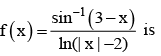Solution: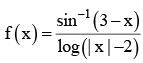Let g(x) = sin -1 (3 - x)
= -1 < 3 - x < 1
The domain of g(x) is [2, 4] and let h(x) = log(|x| - 2)
⇒ | x | -2 > 0 or  |x| > 2   and | x | -2 ≠ 1 ⇒ | x | ≠ 3 ⇒ x ≠ ±3
⇒ x < -2  or  x > 2
⇒ (-∞, -2) ∪ (2, ∞)
Therefore, the domain of f(x)
= (2,4] - {3} = (2, 3) ∪ (3, 4]

QUESTION: 2

### The domain of f (x) = log | logx | is

Solution:

f(x) = log|logx|, f(x) is defined if |log x| > 0 and x > 0,
i.e., if x > 0 and x ≠ 1
(∵ |log x| > 0 if x ≠ 1)
⇒ x ∈ (0,1)∪(1,∞).

QUESTION: 3

### The domain of the function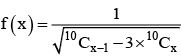contains the points

Solution:

Given function is defined if 10Cx-1 >3  10Cx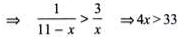Moreover, 10 > x – 1 and 10 > x
⇒ x > 9 but x < 10 ⇒ x = 9, 10.
[∴ x ∈N]

QUESTION: 4

The range of the function f (x) = cot-1 (2x- x2) is

Solution:

Let y = cot-1 (2x - x2)
⇒ y = cot-1 {1- (x - 1)2}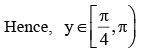QUESTION: 5

The range of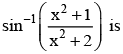Solution: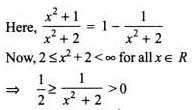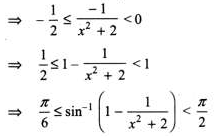QUESTION: 6

The function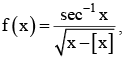where [x] denotes the greatest integer less than or equal to x, is defined for all x ∈

Solution:

The function sec-1 x is defined for all x ∈ R - (-1, 1) and the function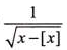is defined for all x ∈ R - Z
So the given function is defined for all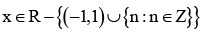QUESTION: 7

The domain of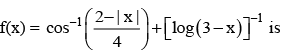Solution: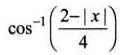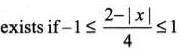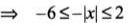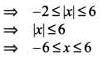The function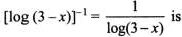defined if 3 - x > and x ≠ 2, i.e., if ≠ 2 and x > 3.
Thus, the domain of the given function is {x| - 6 < x < 6} ∩ {x| x ≠ 2, x < 3} = [-6,2)∪(2,3).

QUESTION: 8

The domain of the function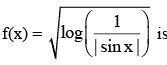Solution: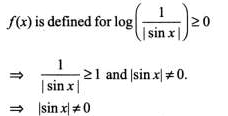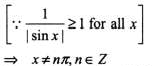Hence, the domain of f(x) = R - (nπ : n ∈ Z}.

QUESTION: 9

The domain of the function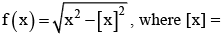greatest integer less than or equal to x, is

Solution:

x2 - [x]2 > 0 ⇒ x2 > [x]2

This is true for all positive values of x and all negative integer x.

QUESTION: 10

If f(x) = ax7 + bx3 + cx – 5; a, b, c are real constants and f (-7) = 7, then the range of f(7) + 17 cos x is

Solution:

f(7) + f(-7) = -10
⇒ f(7) = -17
⇒ f(7) + 17 cos x = -17 + 17 cos x which has the range [-34, 0]

QUESTION: 11

The range of the function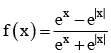Solution: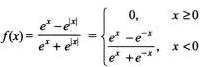Clearly,f(x) is identically zero if x > 0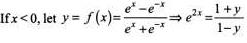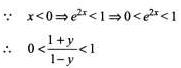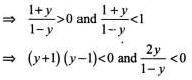⇒ -1 < y < 1and y < 0 or y  > 1
⇒ -1 < y < 0.
Combinning (1) and (2), we get -1 < y < 0
⇒ Range = (-1, 0]

QUESTION: 12

The domain of the function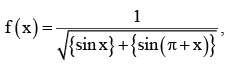where {.} denotes the fractional part, is

Solution: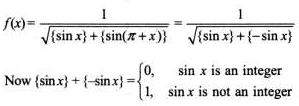For f(x) to get defined {sin x} + {- sin x} ≠ 0
⇒ sin x ≠ integer
⇒ sin x ≠ + 1,0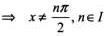Hence the domain is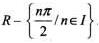QUESTION: 13

The range of the function f defined by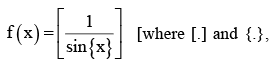respectively, denote the greatest integer and the fractional part] is

Solution:

∴ {x} ∈ [0, 1)
sin {x} ∈ (0, sin 1) as f(x) is defined if sin {x} ≠ 0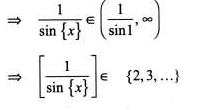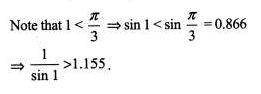QUESTION: 14

Let f(x)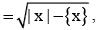(where {.}  denotes the fractional part of x and X, Y are its domain and range, respectively), then

Solution: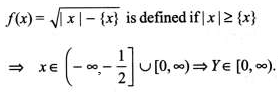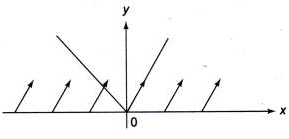QUESTION: 15

The domain of the function :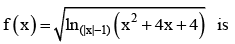Solution:

Case I
0 < |x| - 1 < 1 ⇒ 1 < |x| < 2,then
x2 +4x+4 < 1
⇒ x2+4x+3 < 0
⇒ -3 < x < -1          ......(1)
So x∈ (-2,-1)
(I) Case II
Ix| - 1 > 1 ⇒ |x| > 2, then x2 +4x+4 > 1
⇒ x2+4x+3 > 0
= x > -1 or x < -3
So,x∈ (-∞,-3) ∪(2,∞)           ......(2)
From(1) and (2),x∈ (-∞,-3) ∪(-2,-1) ∪ (2,∞)

QUESTION: 16

The domain of the function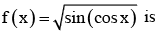Solution:

For f(x) to be defined, cosx > 0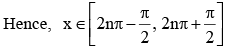QUESTION: 17

The domain of f(x)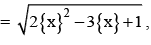where {.} denotes the fractional part in [-1,1], is

Solution:

We must have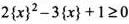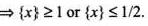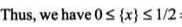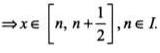QUESTION: 18

The range of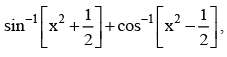where [.] denotes the greater integer  function, is

Solution: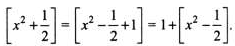Thus, from domain point of view,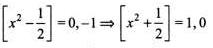⇒ f(x) = sin-1(1) + cos-1 (0) or sin-1 (0) + cos-1 (-1)
⇒ f(x) = {π}

QUESTION: 19

The domain of f(x) = sin -1 [2x2 - 3], where [.] denotes the greatest integer function, is

Solution:

sin−1[2x2 - 1]
We know that the range of sin−1 is [−π/2,π/2] and domain is [−1,1]
−1≤2x2−3≤2
2≤2x2≤5
2x2−2≥0; 2x2−5≤0
x2 ≥1 ; x2≤5/2
∴ xϵ[−(5/2)1/2,−1]⋃[1,(5/2)1/2]

QUESTION: 20

The range of f(x) = [|sin x |+| cos x|] , where [.] denotes the greatest integer function, is

Solution:

Range of |sinx| is [0,1] and |cosx| is [0,1] for all x belongs to R.
So the range of f(x) is also [0,1] as sin0 =! cos0.
So [|sinx| + |cosx|] = 1 as per the definition of greatest integer function.

QUESTION: 21

If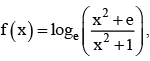then the range of f(x) is

Solution: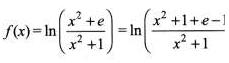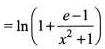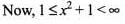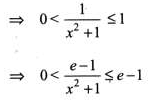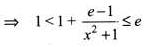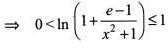Hence, the range is (0, 1].

QUESTION: 22

The range of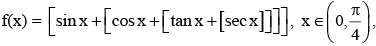where [.] denote the greatest integer function £ x , is

Solution:

Given f(x) = [sinx + [cosx + [tanx+ [secx]]]]
= [sinx + p], where p = [cosx + [tanx+ [sccx]]]
= [sinx] +p, (as p is an integer)
= [sinx] + [cosx + [tanx+ [secx]]]
= [sinx] + [cosx] + [tanx] + [secx]
Now, for x ∈ (0, π/4), sin x ∈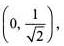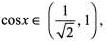tan x ∈ (0, 1), sec x ∈ (1, √2)
⇒[sin x] = 0, [cos x] = 0, [tan x] = 0 and [sec x] = 1
⇒ The range of f(x) is 1,

QUESTION: 23

Let  A = {1, 2, 3} and let R = {(1, 1), (2, 2), (3, 3), (1, 3), (3, 2), (1, 2)}. Then  R is

Solution:

R is reflexive and transitive but not symmetric

QUESTION: 24

Let A = {a, b, c} and let R = {(a, a)(a, b), (b, a)}. Then, R is

Solution:

R is symmetric and transitive but not reflexive

QUESTION: 25

Let A = {1, 2, 3} then total number of relations in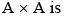Solution:

Total number of relations = 2n2

QUESTION: 26

Let S be the set of all straight lines in a plane. Let R be a relation on S defined by a R b ⇔ a ⊥ b.  Then, R is

Solution:

a ⊥ a is not true. So, R is not reflexive
a ⊥ b and b ⊥ c does not imply a ⊥ c. So, R is not transitive
But, a ⊥ b ⇒ b ⊥ a is always true. So, R is symmetric.

QUESTION: 27

Let S be the set of all real numbers and let R be a relation on S, defined by a R b ⇔ |a – b| < 1. Then, R is

Solution:

(i) |a – a| = 0 < 1 is always true
(ii) a R b ⇒ |a – b| < 1 ⇒ |-(a – b)| < 1  ⇒ |b – a| < 1 ⇒ b R a.  So, R is symmetric.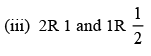But, 2 is not related to 1/2. So, R is not transitive.

QUESTION: 28

Let W denote the words in the English dictionary. Define the relation R by R = {(x, y) ∈ W × W| the words x and y have at least one letter in common}. Then, R is

Solution:

Let W = {CAT, TOY, YOU, ……..)
Clearly, R is reflexive and symmetric but not transitive.
Since, CATRTOY, TOYRYOU ⇒ CATRYOU

QUESTION: 29

Let R = {(3, 3), (6, 6), (9, 9), (3,6), (3, 9), (9, 12), (3,12), (6, 12), (12, 12)}, be a relation on the set A = {3, 6, 9, 12} Then the relation is

Solution:

R is reflexive
∴ (3, 3), (6,6), (9, 9), (12, 12) ∈ R
(6, 12) ∈ R but (12, 6) ∈ R Þ R is not symmetric
R is transitive
∴ (3, 6) ∈ R, (6, 12) ∈ R, (3, 12) ∈ R

QUESTION: 30

Let R be a relation on the set A of ordered pairs of positive integers defined by (x, y)R(u, v) if and only if xv = yu, then

Solution:

Clearly,(x, y) R(x,y),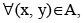since xy = yx. This shows that R is reflexive.
Further, (x, y)R(u, v) ⇒ xv = yu ⇒ uy = vx and hence (u, v)R(x, y).
This shows that R is symmetric. Similarly, (x, y)R(u, v) and (u, v)R(a, b) ⇒ xv = yu and ub = va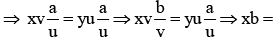ya and hence (x, y)R(a, b). Thus, R is transitive. Thus, R is an equivalent relation.